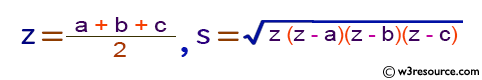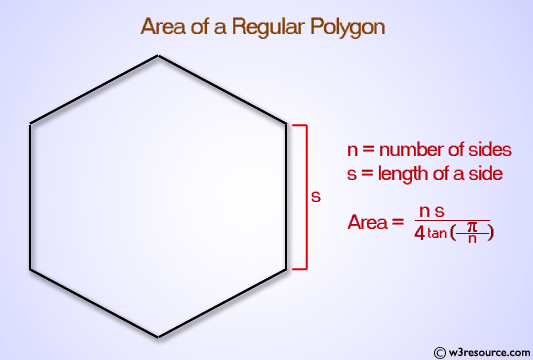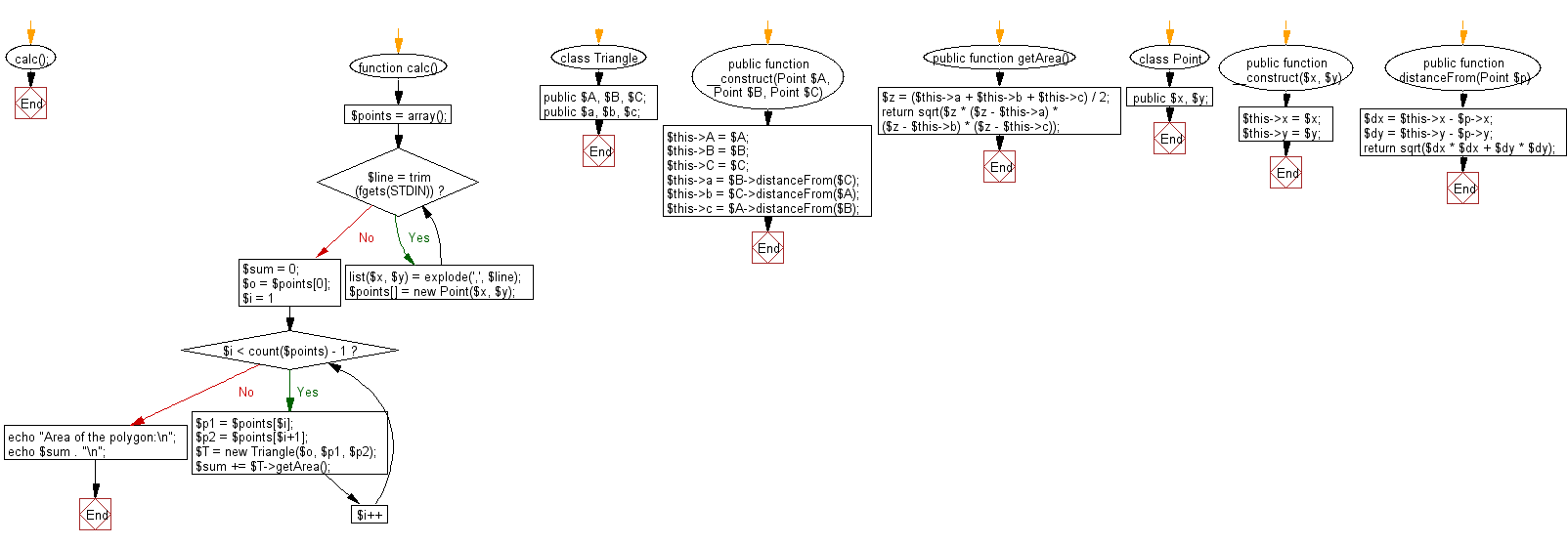﻿ PHP Exercise: Compute the area of the polygon - w3resource

# PHP Exercises: Compute the area of the polygon

## PHP: Exercise-73 with Solution

A convex polygon is a simple polygon in which no line segment between two points on the boundary ever goes outside the polygon. Equivalently, it is a simple polygon whose interior is a convex set. In a convex polygon, all interior angles are less than or equal to 180 degrees, while in a strictly convex polygon all interior angles are strictly less than 180 degrees.
Write a PHP program that compute the area of the polygon . The vertices have the names vertex 1, vertex 2, vertex 3, ... vertex n according to the order of edge connections. However, n is 3 or more and 20 or less. You can also use the following formula to calculate the area S from the lengths a, b, and c of the triangle's three sides.

Input: Multiple character strings are given. One string is given per linePictorial Presentation:Input:
Input is given in the following format.
x1 , y1
x2 , y2
:
xn , yn
xi , yi are real numbers representing the x and y coordinates of vertex i , respectively.

Sample Solution: -

PHP Code:

``````<?php
calc();

function calc() {
\$points = array();
while (\$line = trim(fgets(STDIN))) {
list(\$x, \$y) = explode(',', \$line);
\$points[] = new Point(\$x, \$y);
}
\$sum = 0;
\$o = \$points;
for (\$i = 1; \$i < count(\$points) - 1; \$i++) {
\$p1 = \$points[\$i];
\$p2 = \$points[\$i+1];

\$T = new Triangle(\$o, \$p1, \$p2);
\$sum += \$T->getArea();
}
echo "Area of the polygon:\n";
echo \$sum . "\n";
}

class Triangle {
public \$A, \$B, \$C;
public \$a, \$b, \$c;

public function __construct(Point \$A, Point \$B, Point \$C) {
\$this->A = \$A;
\$this->B = \$B;
\$this->C = \$C;

\$this->a = \$B->distanceFrom(\$C);
\$this->b = \$C->distanceFrom(\$A);
\$this->c = \$A->distanceFrom(\$B);
}

public function getArea() {
\$z = (\$this->a + \$this->b + \$this->c) / 2;
return sqrt(\$z * (\$z - \$this->a) * (\$z - \$this->b) * (\$z - \$this->c));
}
}

class Point {
public \$x, \$y;

public function __construct(\$x, \$y) {
\$this->x = \$x;
\$this->y = \$y;
}

public function distanceFrom(Point \$p) {
\$dx = \$this->x - \$p->x;
\$dy = \$this->y - \$p->y;
return sqrt(\$dx * \$dx + \$dy * \$dy);
}
}
?>
```
```

Sample Input:
1.0, 0.0
0.0, 0.0
1.0, 1.0
2.0, 0.0
-1.0, 1.0

Sample Output:

```Area of the polygon:
1.5```

Flowchart:PHP Code Editor:

Have another way to solve this solution? Contribute your code (and comments) through Disqus.

What is the difficulty level of this exercise?

Test your Programming skills with w3resource's quiz.

﻿

## PHP: Tips of the Day

Returns all elements in an array except for the first one

Example:

```<?php
function tips_tail(\$items)
{
return count(\$items) > 1 ? array_slice(\$items, 1) : \$items;
}

print_r(tips_tail([1, 5, 7]));
?>
```

Output:

```Array
(
 => 5
 => 7
)
```